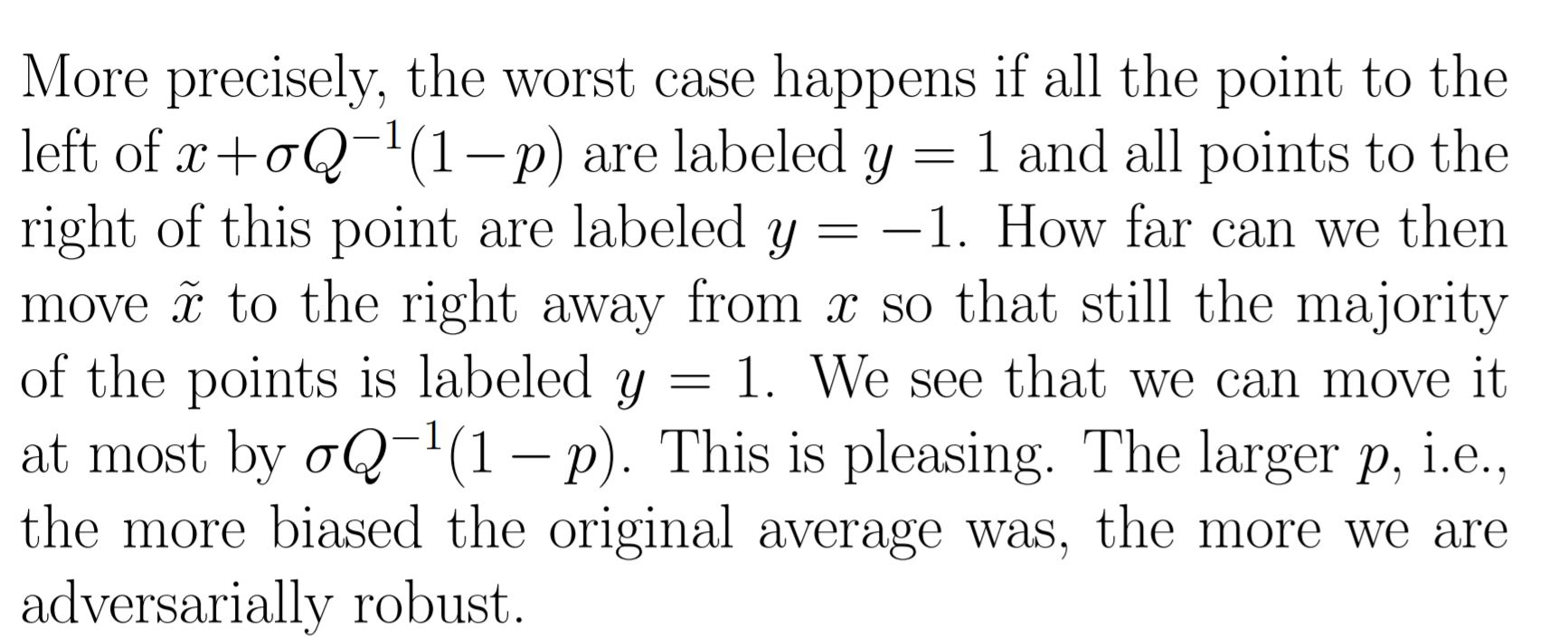### randomized smoothing

Hello,

I don't understand this explanation. So we have a point x that has expected value of classifier f(x+z) bigger than 1/2. Then it says all points on the left are + all on right are negative. In that case will Expected value be 1/2 exactly?

Secondly, I don't understand what Q^-1 is and what exactly we are trying to do do in this example, what is our final classifier? why is it better than f?

thank youPage 1 of 1